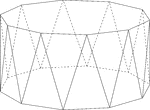Decagonal Antiprism

Illustration of a decagonal antiprism. An antiprism is formed by having two parallel congruent bases…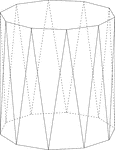Decagonal Antiprism

Illustration of a decagonal antiprism. An antiprism is formed by having two parallel congruent bases…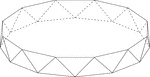Dodecagonal Antiprism

Illustration of a dodecagonal antiprism. An antiprism is formed by having two parallel congruent bases…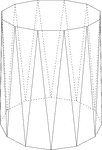Dodecagonal Antiprism

Illustration of a dodecagonal antiprism. An antiprism is formed by having two parallel congruent bases…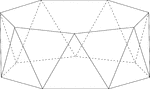Heptagonal/Septagonal Antiprism

Illustration of a heptagonal, or sometimes known as a septagonal antiprism. An antiprism is formed by…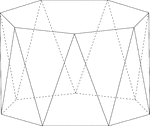Heptagonal/Septagonal Antiprism

Illustration of a heptagonal, or sometimes known as a septagonal antiprism. An antiprism is formed by…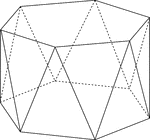Hexagonal Antiprism

Illustration of a hexagonal antiprism. An antiprism is formed by having two parallel congruent bases…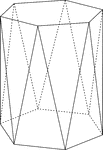Hexagonal Antiprism

Illustration of a hexagonal antiprism. An antiprism is formed by having two parallel congruent bases…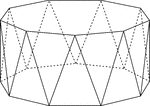Nonagonal Antiprism

Illustration of a nonagonal antiprism. An antiprism is formed by having two parallel congruent bases…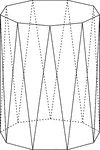Nonagonal Antiprism

Illustration of a nonagonal antiprism. An antiprism is formed by having two parallel congruent bases…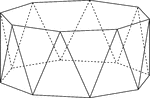Octagonal Antiprism

Illustration of an octagonal antiprism. An antiprism is formed by having two parallel congruent bases…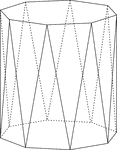Octagonal Antiprism

Illustration of an octagonal antiprism. An antiprism is formed by having two parallel congruent bases…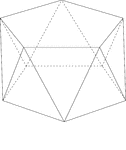Pentagonal Antiprism

Illustration of a pentagonal antiprism. An antiprism is formed by having two parallel congruent bases…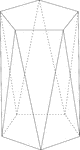Pentagonal Antiprism

Illustration of a pentagonal antiprism. An antiprism is formed by having two parallel congruent bases…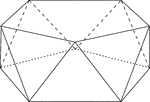Pentagonal Antiprism

Illustration of a pentagonal antiprism. An antiprism is formed by having two parallel congruent bases…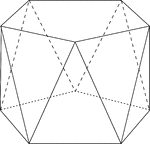Pentagonal Antiprism

Illustration of a pentagonal antiprism. An antiprism is formed by having two parallel congruent bases…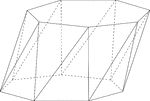Skewed Heptagonal/Septagonal Antiprism

Illustration of a skewed (non-right) heptagonal, or sometimes known as a septagonal antiprism. An antiprism…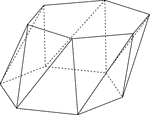Skewed Hexagonal Antiprism

Illustration of a skewed (non-right) hexagonal antiprism. An antiprism is formed by having two parallel…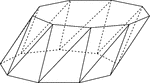Skewed Nonagonal Antiprism

Illustration of a skewed (non-right) nonagonal antiprism. An antiprism is formed by having two parallel…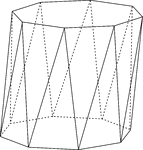Skewed Octagonal Antiprism

Illustration of a skewed (non-right) octagonal antiprism. An antiprism is formed by having two parallel…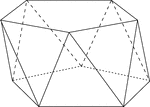Skewed Pentagonal Antiprism

Illustration of a skewed (non-right) pentagonal antiprism. An antiprism is formed by having two parallel…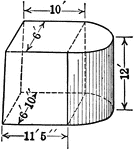Composite Figure of Quadrilateral Frustum With Half of a Cylinder Attached

An illustration of a composite figure made up of a quadrilateral frustum and half of a cylinder. Frustum…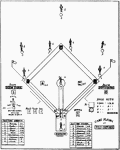Baseball Game Illustration

This is an illustration of a baseball game. Baseball is played on a large field that has four bases…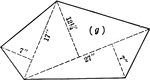Pentagon With Triangular Sections For Area

Pentagon with dimensions labeled. Pentagon can be used to calculate area by calculating individual triangles…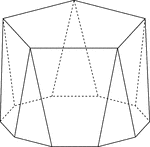Polyhedron With Pentagon Bases

Illustration of a pentagonal polyhedron that is formed by having two parallel congruent pentagonal bases…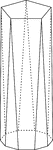Polyhedron With Pentagon Bases

Illustration of a pentagonal polyhedron that is formed by having two parallel congruent pentagonal bases…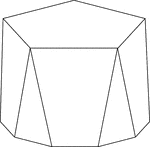Polyhedron With Pentagon Bases

Illustration of a pentagonal polyhedron that is formed by having two parallel congruent pentagonal bases…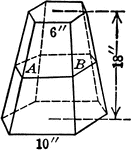Pyramid Frustum With Hexagonal Bases and 6 inch and 10 inch Sides

An illustration of a pyramid with the top cut off by a plane parallel to the base. The remaining part…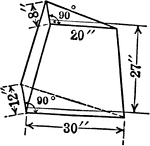Pyramid Frustum With Triangular Bases and Height of 27 inches

An illustration of a pyramid with the top cut off by a plane parallel to the base. The remaining part…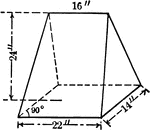Pyramid Frustum With Triangular Bases and Height of 27 inches

An illustration of a pyramid with the top cut off by a plane parallel to the base. The remaining part…Sphere With 8-inch Diameter Cut by Planes

An 8-inch sphere cut by parallel planes, one 2 inches from center and the other 6 inches from center.…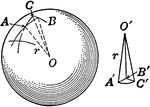Sphere Cut Into Pyramids.

An illustration of a sphere cut into polygons as bases with their vertices at the center of sphere.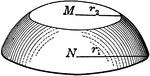Zones or Segments of Spheres

An illustration of a zone of a sphere. A zone occurs when a sphere is cut by parallel planes that are…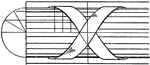Modern Enrichment Torus Moulding

The modern enrichment torus moulding is a bundle of round rods with ribbons twisted around. These designs…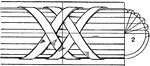Modern Enrichment Torus Moulding

The modern enrichment torus moulding is a bundle of round rods with ribbons twisted around. These designs…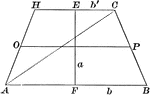Area of a Trapezoid

Illustration of a trapezoid with altitude a and bases b and b' used to demonstrate that the area is…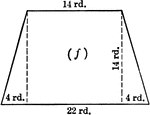Trapezoid With Dimensions

Trapezoid with dimensions labeled. Trapezoid can be used to calculate area.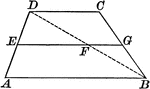Trapezoid With Median

Illustration of a trapezoid, with the median drawn. The median of a trapezoid is parallel to the bases,…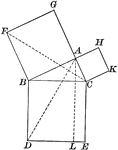Right Triangle

Illustration of a right triangle used to show the Pythagorean Theorem (the square of the hypotenuse…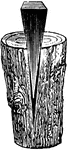Wedge Splitting Wood

"[A wedge] is simply a movable inclined plane, or two such planes united a their bases." -Avery 1895